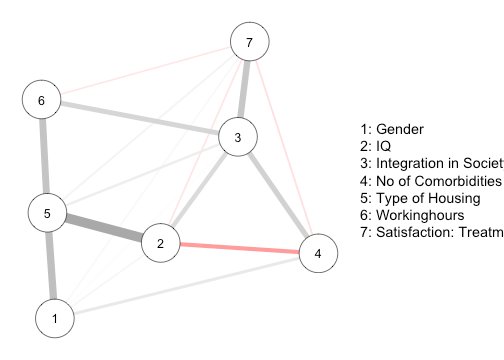Want to share your content on R-bloggers? click here if you have a blog, or here if you don't.

Interpreting statistical network models typically involves interpreting individual edge parameters. If the network model is a Gaussian Graphical Model (GGM), the interpretation is relatively simple: the pairwise interaction parameters are partial correlations, which indicate conditional linear relationships and vary from -1 to 1. Using the standard deviations of the two involved variables, the partial correlation can also be transformed into a linear regression coefficient (see for example here). However, when studying interactions involving categorical variables, such as in an Ising model or a Mixed Graphical Model (MGM), the parameters are not limited to a certain range and their interpretation is less intuitive. In these situations it may be helpful to report the interactions between variables in terms of odds ratios.

### Odds Ratios

Odds Ratios are made up of odds, which are themselves a ratio of probabilities

$$\text{Odds} = \frac{P(X_1=1)}{P(X_1=0)}.$$ Since we chose to put $P(X_1=1)$ in the numerator, we interpret these odds as the “odds being in favor of $X_1=1$”. For example, if $X_1$ is the symptom sleep problems which takes the values 0 (no sleep problems) with probability 0.75 and the value 1 (sleep problems) with probability 0.25, then the odds of having sleep problems are 1 to 4.

However, these odds may be different in different circumstances. Let’s say these circumstances are captured by variable $X_2$ which takes values in ${0,1}$. In our example, those circumstances could be whether you live next to a busy street (1) or not (0). If the odds indeed depend on $X_2$ then we have

$\text{Odds}_{X_2=1} = \frac{P(X_1=1 \mid X_2=1)}{P(X_1=0 \mid X_2=1)} \neq \frac{P(X_1=1 \mid X_2=0)}{P(X_1=0 \mid X_2=0)} = \text{Odds}_{X_2=0} .$

A way to quantify the degree to which the odds are different depending whether we set $X_2=1$ or $X_2=0$ is to divide the odds in those two situations

$\text{Odds Ratio} = \frac{\text{Odds}_{X_2=1}}{\text{Odds}_{X_2=0}} ,$

which gives rise to an odds ratio (OR).

How do we interpret this odds ratio? If the OR is equal to 1, then $X_2$ has no influence on the odds between $P(X_1=1)$ and $P(X_1=0)$; if OR > 1, $X_2=1$ increases the odds compared to $X_2=0$; and if OR < 1, $X_2=1$ decreases the odds compared to $X_2=0$. In our example from above, an OR = 4 would imply that the odds of sleep problems (vs. no sleep problems) are four times larger when living next to a busy street (vs. not living next to a busy street).

In the remainder of this blog post, I will illustrate how to compute such odds ratios based on MGMs estimated with the R-package mgm.

We use a data set on Autism Spectrum Disorder (ASD) which contains $n=3521$ observations of seven variables, gender (1 = male, 2 = female), IQ (continuous), Integration in Society (3 categories ordinal), Number of comorbidities (count), Type of housing (1 = supervised, 2 = unsupervised), working hours (continuous), and satisfaction with treatment (continuous).

For more details on this data set have a look at this previous blog post.

### Fitting MGM

We model gender, integration in society and type of housing as categorical variables (with 2, 3 and 2 categories), and all remaining variables as Gaussian variables

and we visualize the dependencies of the resulting model using the qqgraph package:### Example Calculation of Odds Ratio

We consider the simplest possible example of calculating the OR involving two binary variables. Note that we calculate the OR between two variables within a multivariate model. This means that the OR is conditional on all other variables in the model which implies that it can be different from the OR calculated based on those two variables alone (specifically, this will always happen when at least one additional variable is connected to both binary variables.)

Some of you might know that there is a simple relationship between the OR and the coefficients in logistic regression. Since multinomial regression with two outcomes is equivalent to logistic regression, we could use this simple rule in this specific example. Here, however, we start out with the definition of OR and show how to calculate it in the general case of $\geq 2$ categories. Along the way, we’ll also derive the simple relationship between parameters and ORs for the binary case.

In our data set we use the two binary variables type of housing and gender for illustration. Specifically, we look at how the odds of type of housing change as a function of gender. Corresponding to the column numbers of the two variables, let $X_5$ be type of housing, and $X_1$ gender.

The definition of ORs above shows that we need to calculate four conditional probabilities. We first calculate $P(X_5=1 \mid X_1=0)$ and $P(X_5=0 \mid X_1=0)$ in the numerator. To compute these probabilities, we need the estimated parameters of the multinomial regression on $X_5$. In the standard parameterization of multinomial regression one of the response categories serves as the reference category (see here). The regularization used within the mgm package allows more direct parameterization in which the probability of each response category can be modeled directly (for details see Chapter 4 in this paper on the glmnet package). We therefore get a set of parameters for each response category.

### Summary

Starting out with the definition of odds ratios, I showed how to compute them in a general setting and how to compute them from the output of the mgm-package. We looked into whether the OR depends on the specific values at which we fix the other variables (it doesn’t). Proving this fact revealed a simpler formula for calculating ORs for the special case of having a binary response variable. Finally, we considered predicted probabilities as an alternative for ORs.

I would like to thank Oisín Ryan for his feedback on this blog post.